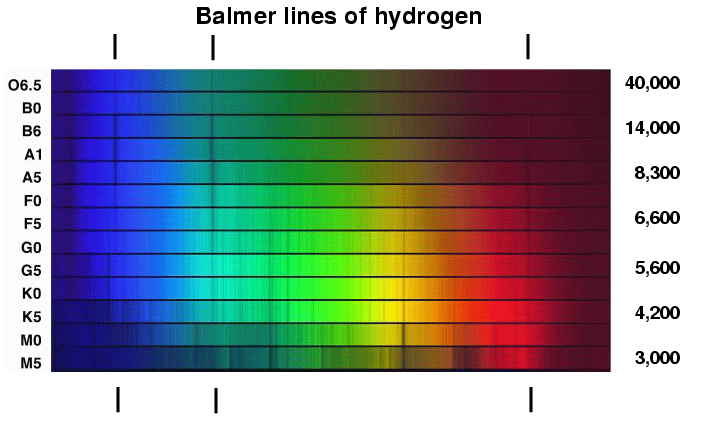## Monday, February 9, 2009

### Dumb + Time = DumberI was just looking for something on my computer and I came across an old astronomy test I took last year and I had one of those moment when I realized that I no longer know how to do the things I used to know how to do.

Or, I suppose, I may never have known how to do them. I think I got this answer wrong.

Question #9. The hydrogen Balmer line of quasar 3C273 is observed to have a wavelength of 563.9 nm. The laboratory hydrogen Balmer line is known to be 486.1 nm. What is the speed at which this quasar is moving away from us expressed in meters per second only? Remember that all calculations require an explanation of your thought processes. Just a single numerical answer with out explanation may not be given any credit.

This question refers to redshift and the formula for calculating redshift is the change in wavelength divided by the unshifted wavelength, which in this case would be the hydrogen Balmer line of quasar 3C273, less the laboratory Balmer line, over the laboratory Balmer line:

Change in wavelength = (563.9 nm - 486.1 nm) = 77.8

So,
Change in wavelength = 77.8 nm
Unshifted wavelength = 486.1 nm = 0.16

Then to get the distance in meters per second, we have to go back to the fact that radial velocity divided by the speed of light is going to be equal to the change in wavelength divided by the unshifted wavelength. So …

Change in wavelength = 77.8 nm
Unshifted wavelength = 486.1 nm = 0.16 multiplied by the speed of light (c)

C is usually expressed as 300,000 km/s
So …
0.16(300,000 km/s) = 48,000 km/s

And finally, to get the answer in meters per second, we multiply by 1000 (the number of meters in a kilometer). So …

1000(48,000 km/s) = 48,000,000 m/s

Quasar 3C273 is moving away from us at a speed of 48 million meters per second.